Cover complex

## Classes

class  Gudhi::cover_complex::Cover_complex< Point >
Cover complex data structure. More...

## Detailed Description

Visualizations of the simplicial complexes can be done with either neato (from graphviz), geomview, KeplerMapper. Input point clouds are assumed to be OFF files

# Covers

Nerves and Graph Induced Complexes require a cover C of the input point cloud P, that is a set of subsets of P whose union is P itself. Very often, this cover is obtained from the preimage of a family of intervals covering the image of some scalar-valued function f defined on P. This family is parameterized by its resolution, which can be either the number or the length of the intervals, and its gain, which is the overlap percentage between consecutive intervals (ordered by their first values).

# Nerves

## Nerve definition

Assume you are given a cover C of your point cloud P. Then, the Nerve of this cover is the simplicial complex that has one k-simplex per k-fold intersection of cover elements. See also Wikipedia .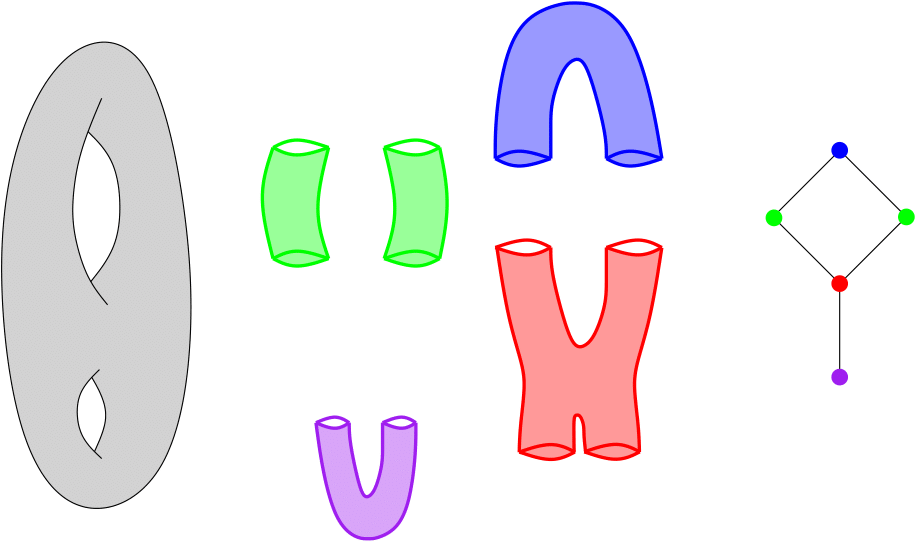Nerve of a double torus

## Example

This example builds the Nerve of a point cloud sampled on a 3D human shape (human.off). The cover C comes from the preimages of intervals (10 intervals with gain 0.3) covering the height function (coordinate 2), which are then refined into their connected components using the triangulation of the .OFF file.

/* This file is part of the Gudhi Library - https://gudhi.inria.fr/ - which is released under MIT.
* See file LICENSE or go to https://gudhi.inria.fr/licensing/ for full license details.
* Author(s): Mathieu Carrière
*
*
* Modification(s):
* - YYYY/MM Author: Description of the modification
*/
#include <gudhi/GIC.h>
#include <string>
#include <vector>
void usage(int nbArgs, char *const progName) {
std::cerr << "Error: Number of arguments (" << nbArgs << ") is not correct\n";
std::cerr << "Usage: " << progName << " filename.off coordinate resolution gain [-v] \n";
std::cerr << " i.e.: " << progName << " ../../data/points/human.off 2 10 0.3 -v \n";
exit(-1); // ----- >>
}
int main(int argc, char **argv) {
if ((argc != 5) && (argc != 6)) usage(argc, argv);
using Point = std::vector<float>;
std::string off_file_name(argv);
int coord = atoi(argv);
int resolution = atoi(argv);
double gain = atof(argv);
bool verb = 0;
if (argc == 6) verb = 1;
// --------------------------------
// Init of a Nerve from an OFF file
// --------------------------------
SC.set_verbose(verb);
if (!check) {
std::clog << "Incorrect OFF file." << std::endl;
} else {
SC.set_type("Nerve");
SC.set_gain(gain);
SC.write_info();
SC.create_complex(stree);
SC.compute_PD();
// ----------------------------------------------------------------------------
// Display information about the graph induced complex
// ----------------------------------------------------------------------------
if (verb) {
std::clog << "Nerve is of dimension " << stree.dimension() << " - " << stree.num_simplices() << " simplices - "
<< stree.num_vertices() << " vertices." << std::endl;
std::clog << "Iterator on Nerve simplices" << std::endl;
for (auto f_simplex : stree.filtration_simplex_range()) {
for (auto vertex : stree.simplex_vertex_range(f_simplex)) {
std::clog << vertex << " ";
}
std::clog << std::endl;
}
}
}
return 0;
}
Simplex Tree data structure for representing simplicial complexes.
Definition: Simplex_tree.h:79
Filtration_simplex_range const & filtration_simplex_range(Indexing_tag=Indexing_tag())
Returns a range over the simplices of the simplicial complex, in the order of the filtration.
Definition: Simplex_tree.h:271
Simplex_vertex_range simplex_vertex_range(Simplex_handle sh) const
Returns a range over the vertices of a simplex.
Definition: Simplex_tree.h:282
size_t num_vertices() const
Returns the number of vertices in the complex.
Definition: Simplex_tree.h:572
int dimension(Simplex_handle sh)
Returns the dimension of a simplex.
Definition: Simplex_tree.h:600
size_t num_simplices()
returns the number of simplices in the simplex_tree.
Definition: Simplex_tree.h:578
Cover complex data structure.
Definition: GIC.h:87
void set_resolution_with_interval_number(int reso)
Sets a number of intervals from a value stored in memory.
Definition: GIC.h:599
Persistence_diagram compute_PD()
Computes the extended persistence diagram of the complex.
Definition: GIC.h:1139
void create_complex(SimplicialComplex &complex)
Creates the simplicial complex.
Definition: GIC.h:1292
void set_type(const std::string &t)
Specifies whether the type of the output simplicial complex.
Definition: GIC.h:176
void find_simplices()
Computes the simplices of the simplicial complex.
Definition: GIC.h:1306
void set_cover_from_function()
Creates a cover C from the preimages of the function f.
Definition: GIC.h:611
void write_info()
Creates a .txt file called SC.txt describing the 1-skeleton, which can then be plotted with e....
Definition: GIC.h:1052
void set_gain(double g=0.3)
Sets a gain from a value stored in memory (default value 0.3).
Definition: GIC.h:605
void set_function_from_coordinate(int k)
Creates the function f from the k-th coordinate of the point cloud P.
Definition: GIC.h:514
void set_color_from_coordinate(int k=0)
Computes the function used to color the nodes of the simplicial complex from the k-th coordinate.
Definition: GIC.h:975
void set_graph_from_OFF()
Creates a graph G from the triangulation given by the input .OFF file.
Definition: GIC.h:332
Reads and stores the input point cloud from .(n)OFF file.
Definition: GIC.h:232
void set_verbose(bool verb=false)
Specifies whether the program should display information or not.
Definition: GIC.h:184

When launching:

$> ./Nerve ../../data/points/human.off 2 10 0.3 -v the program output is: Min function value = -0.979672 and Max function value = 0.816414 Interval 0 = [-0.979672, -0.761576] Interval 1 = [-0.838551, -0.581967] Interval 2 = [-0.658942, -0.402359] Interval 3 = [-0.479334, -0.22275] Interval 4 = [-0.299725, -0.0431415] Interval 5 = [-0.120117, 0.136467] Interval 6 = [0.059492, 0.316076] Interval 7 = [0.239101, 0.495684] Interval 8 = [0.418709, 0.675293] Interval 9 = [0.598318, 0.816414] Computing preimages... Computing connected components... .txt generated. It can be visualized with e.g. python KeplerMapperVisuFromTxtFile.py and firefox. 5 interval(s) in dimension 0: [-0.909111, 0.00817529] [-0.171433, 0.367392] [-0.171433, 0.367392] [-0.909111, 0.745853] 0 interval(s) in dimension 1: Nerve is of dimension 1 - 41 simplices - 21 vertices. Iterator on Nerve simplices 1 0 4 4 0 2 2 1 8 8 2 5 5 4 9 9 8 13 13 5 14 14 9 19 19 13 25 32 20 32 20 33 33 25 26 26 14 26 19 42 42 26 34 34 33 27 27 20 35 35 27 35 34 42 35 44 44 35 54 54 44 The program also writes a file ../../data/points/human_sc.txt. The first three lines in this file are the location of the input point cloud and the function used to compute the cover. The fourth line contains the number of vertices nv and edges ne of the Nerve. The next nv lines represent the vertices. Each line contains the vertex ID, the number of data points it contains, and their average color function value. Finally, the next ne lines represent the edges, characterized by the ID of their vertices. Using KeplerMapper, one can obtain the following visualization: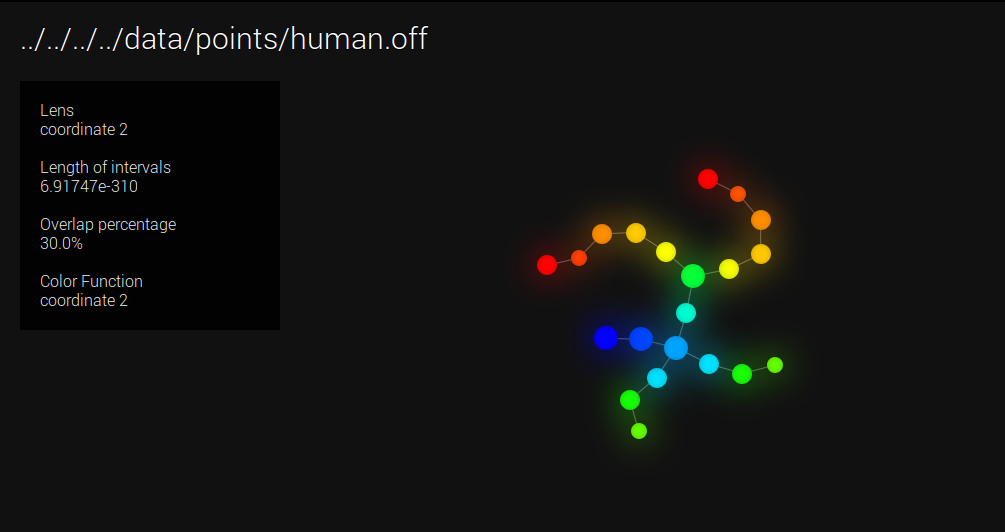Visualization with KeplerMapper # Graph Induced Complexes (GIC) ## GIC definition Again, assume you are given a cover C of your point cloud P. Moreover, assume you are also given a graph G built on top of P. Then, for any clique in G whose nodes all belong to different elements of C, the GIC includes a corresponding simplex, whose dimension is the number of nodes in the clique minus one. See  for more details.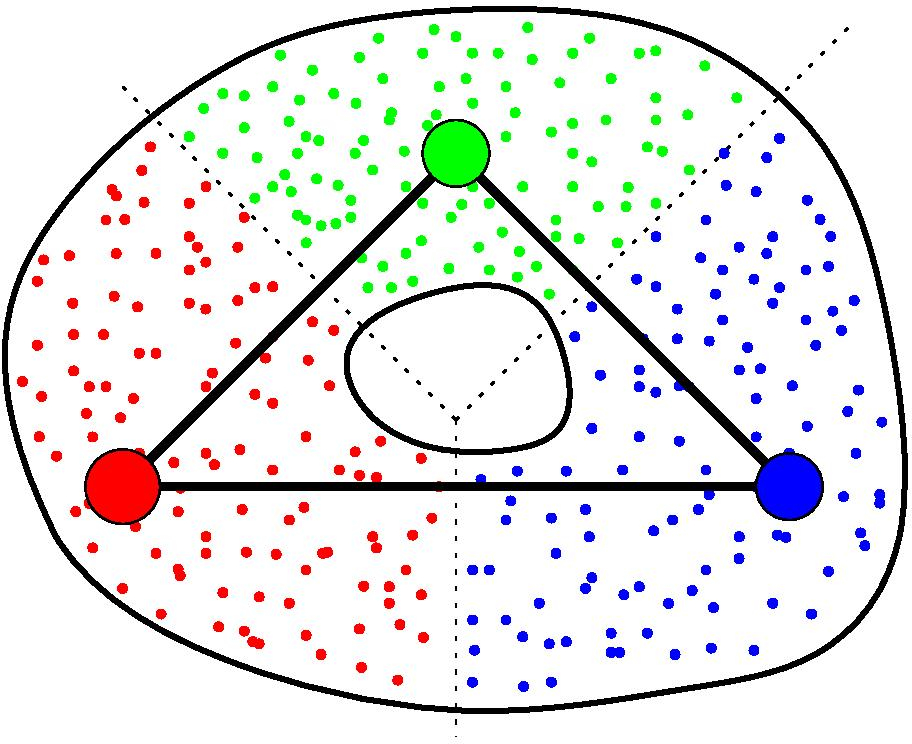GIC of a point cloud. ## Example with cover from Voronoï This example builds the GIC of a point cloud sampled on a 3D human shape (human.off). We randomly subsampled 100 points in the point cloud, which act as seeds of a geodesic Voronoï diagram. Each cell of the diagram is then an element of C. The graph G (used to compute both the geodesics for Voronoï and the GIC) comes from the triangulation of the human shape. Note that the resulting simplicial complex is in dimension 3 in this example. /* This file is part of the Gudhi Library - https://gudhi.inria.fr/ - which is released under MIT. * See file LICENSE or go to https://gudhi.inria.fr/licensing/ for full license details. * Author(s): Mathieu Carrière * * Copyright (C) 2017 Inria * * Modification(s): * - YYYY/MM Author: Description of the modification */ #include <gudhi/GIC.h> #include <string> #include <vector> void usage(int nbArgs, char *const progName) { std::cerr << "Error: Number of arguments (" << nbArgs << ") is not correct\n"; std::cerr << "Usage: " << progName << " filename.off N [-v] \n"; std::cerr << " i.e.: " << progName << " ../../data/points/human.off 100 -v \n"; exit(-1); // ----- >> } int main(int argc, char **argv) { if ((argc != 3) && (argc != 4)) usage(argc, argv); using Point = std::vector<float>; std::string off_file_name(argv); int m = atoi(argv); bool verb = 0; if (argc == 4) verb = 1; // ---------------------------------------------------------------------------- // Init of a graph induced complex from an OFF file // ---------------------------------------------------------------------------- GIC.set_verbose(verb); bool check = GIC.read_point_cloud(off_file_name); if (!check) { std::clog << "Incorrect OFF file." << std::endl; } else { GIC.set_type("GIC"); GIC.plot_OFF(); GIC.create_complex(stree); // ---------------------------------------------------------------------------- // Display information about the graph induced complex // ---------------------------------------------------------------------------- if (verb) { std::clog << "Graph induced complex is of dimension " << stree.dimension() << " - " << stree.num_simplices() << " simplices - " << stree.num_vertices() << " vertices." << std::endl; std::clog << "Iterator on graph induced complex simplices" << std::endl; for (auto f_simplex : stree.filtration_simplex_range()) { for (auto vertex : stree.simplex_vertex_range(f_simplex)) { std::clog << vertex << " "; } std::clog << std::endl; } } } return 0; } Compute the Euclidean distance between two Points given by a range of coordinates.... Definition: distance_functions.h:32 void set_cover_from_Voronoi(Distance distance, int m=100) Creates the cover C from the Voronoï cells of a subsampling of the point cloud. Definition: GIC.h:873 void plot_OFF() Creates a .off file called SC.off for 3D visualization, which contains the 2-skeleton of the GIC.... Definition: GIC.h:1091 When launching:$> ./VoronoiGIC ../../data/points/human.off 700 -v

the program outputs SC.off. Using e.g.

$> geomview ../../data/points/human_sc.off one can obtain the following visualization: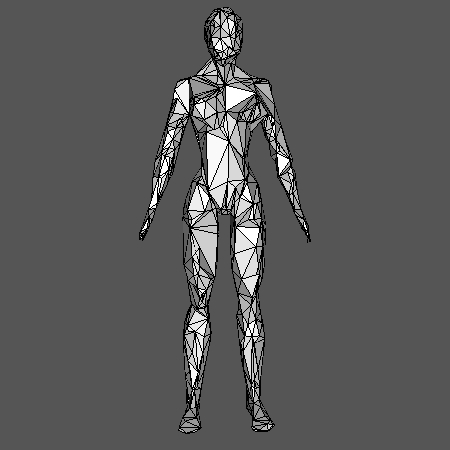Visualization with Geomview ## Functional GIC If one restricts to the cliques in G whose nodes all belong to preimages of consecutive intervals (assuming the cover of the height function is minimal, i.e. no more than two intervals can intersect at a time), the GIC is of dimension one, i.e. a graph. We call this graph the functional GIC. See  for more details. ## Example Functional GIC comes with automatic selection of the Rips threshold, the resolution and the gain of the function cover. See  for more details. In this example, we compute the functional GIC of a Klein bottle embedded in R^5, where the graph G comes from a Rips complex with automatic threshold, and the cover C comes from the preimages of intervals covering the first coordinate, with automatic resolution and gain. Note that automatic threshold, resolution and gain can be computed as well for the Nerve. /* This file is part of the Gudhi Library - https://gudhi.inria.fr/ - which is released under MIT. * See file LICENSE or go to https://gudhi.inria.fr/licensing/ for full license details. * Author(s): Mathieu Carrière * * Copyright (C) 2017 Inria * * Modification(s): * - YYYY/MM Author: Description of the modification */ #include <gudhi/GIC.h> #include <string> #include <vector> void usage(int nbArgs, char *const progName) { std::cerr << "Error: Number of arguments (" << nbArgs << ") is not correct\n"; std::cerr << "Usage: " << progName << " filename.off coordinate [-v] \n"; std::cerr << " i.e.: " << progName << " ../../data/points/human.off 2 -v \n"; exit(-1); // ----- >> } int main(int argc, char **argv) { if ((argc != 3) && (argc != 4)) usage(argc, argv); using Point = std::vector<float>; std::string off_file_name(argv); int coord = atoi(argv); bool verb = 0; if (argc == 4) verb = 1; // ----------------------------------------- // Init of a functional GIC from an OFF file // ----------------------------------------- GIC.set_verbose(verb); bool check = GIC.read_point_cloud(off_file_name); if (!check) { std::clog << "Incorrect OFF file." << std::endl; } else { GIC.set_type("GIC"); GIC.set_gain(); GIC.plot_DOT(); GIC.create_complex(stree); // -------------------------------------------- // Display information about the functional GIC // -------------------------------------------- if (verb) { std::clog << "Coordinate GIC is of dimension " << stree.dimension() << " - " << stree.num_simplices() << " simplices - " << stree.num_vertices() << " vertices." << std::endl; std::clog << "Iterator on coordinate GIC simplices" << std::endl; for (auto f_simplex : stree.filtration_simplex_range()) { for (auto vertex : stree.simplex_vertex_range(f_simplex)) { std::clog << vertex << " "; } std::clog << std::endl; } } } return 0; } double set_automatic_resolution() Computes the optimal length of intervals (i.e. the smallest interval length avoiding discretization a... Definition: GIC.h:552 void plot_DOT() Creates a .dot file called SC.dot for neato (part of the graphviz package) once the simplicial comple... Definition: GIC.h:1004 double set_graph_from_automatic_rips(Distance distance, int N=100) Creates a graph G from a Rips complex whose threshold value is automatically tuned with subsampling—s... Definition: GIC.h:439 void compute_distribution(unsigned int N=100) Computes bootstrapped distances distribution. Definition: GIC.h:1202 double compute_p_value() Computes the p-value, i.e. the opposite of the confidence level of the largest bottleneck distance pr... Definition: GIC.h:1273 When launching:$> ./CoordGIC ../../data/points/KleinBottle5D.off 0 -v

the program outputs SC.dot. Using e.g.

$> neato SC.dot -Tpdf -o SC.pdf one can obtain the following visualization: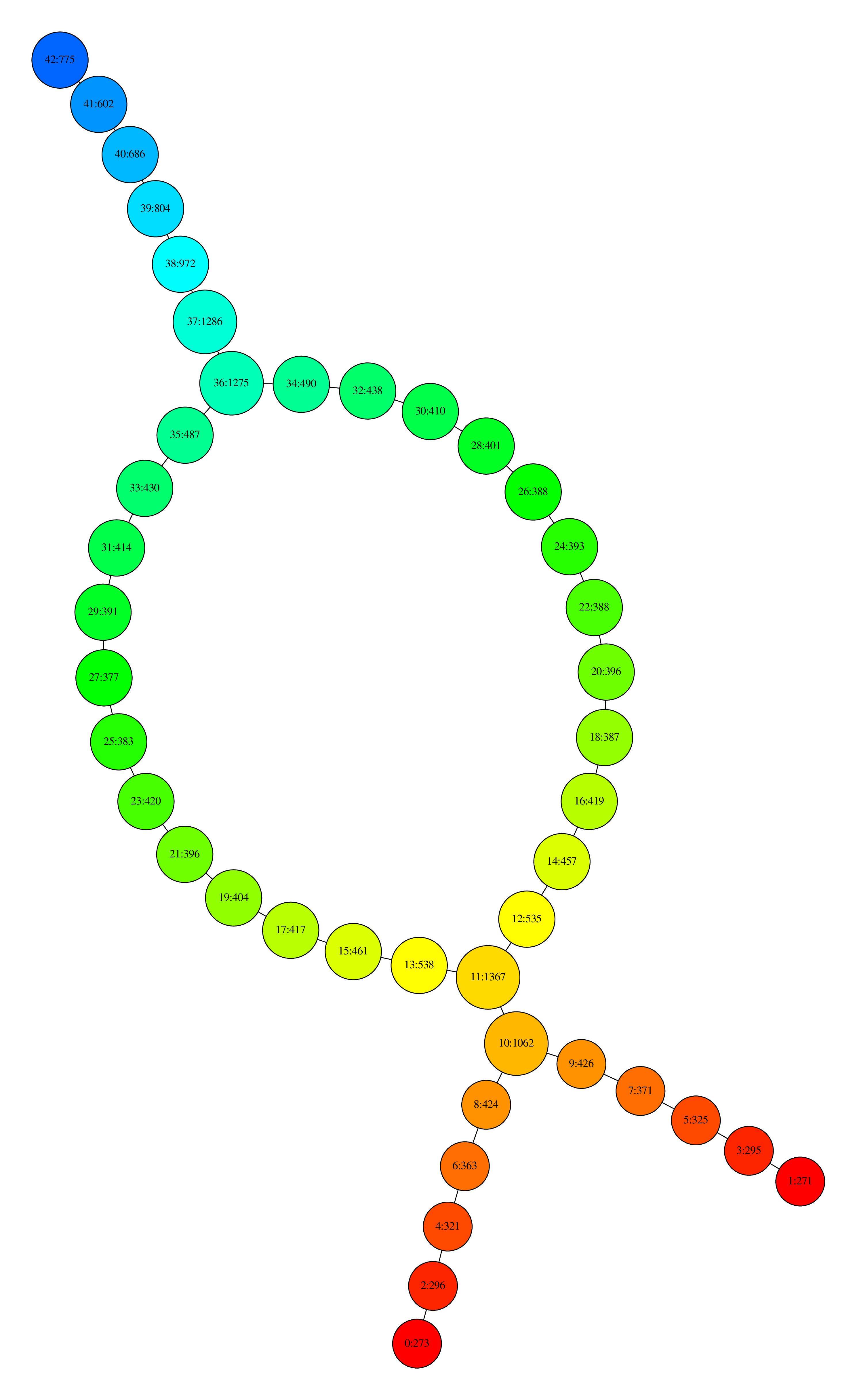Visualization with Neato where nodes are colored by the filter function values and, for each node, the first number is its ID and the second is the number of data points that its contain. We also provide an example on a set of 72 pictures taken around the same object (lucky_cat.off). The function is now the first eigenfunction given by PCA, whose values are written in a file (lucky_cat_PCA1). Threshold, resolution and gain are automatically selected as before. /* This file is part of the Gudhi Library - https://gudhi.inria.fr/ - which is released under MIT. * See file LICENSE or go to https://gudhi.inria.fr/licensing/ for full license details. * Author(s): Mathieu Carrière * * Copyright (C) 2017 Inria * * Modification(s): * - YYYY/MM Author: Description of the modification */ #include <gudhi/GIC.h> #include <string> #include <vector> void usage(int nbArgs, char *const progName) { std::cerr << "Error: Number of arguments (" << nbArgs << ") is not correct\n"; std::cerr << "Usage: " << progName << " filename.off function [-v] \n"; std::cerr << " i.e.: " << progName << " ../../data/points/COIL_database/lucky_cat.off " "../../data/points/COIL_database/lucky_cat_PCA1 -v \n"; exit(-1); // ----- >> } int main(int argc, char **argv) { if ((argc != 3) && (argc != 4)) usage(argc, argv); using Point = std::vector<float>; std::string off_file_name(argv); std::string func_file_name = argv; bool verb = 0; if (argc == 4) verb = 1; // ----------------------------------------- // Init of a functional GIC from an OFF file // ----------------------------------------- GIC.set_verbose(verb); bool check = GIC.read_point_cloud(off_file_name); if (!check) { std::clog << "Incorrect OFF file." << std::endl; } else { GIC.set_type("GIC"); GIC.set_color_from_file(func_file_name); GIC.set_function_from_file(func_file_name); GIC.set_gain(); GIC.plot_DOT(); GIC.create_complex(stree); // -------------------------------------------- // Display information about the functional GIC // -------------------------------------------- if (verb) { std::clog << "Functional GIC is of dimension " << stree.dimension() << " - " << stree.num_simplices() << " simplices - " << stree.num_vertices() << " vertices." << std::endl; std::clog << "Iterator on functional GIC simplices" << std::endl; for (auto f_simplex : stree.filtration_simplex_range()) { for (auto vertex : stree.simplex_vertex_range(f_simplex)) { std::clog << vertex << " "; } std::clog << std::endl; } } } return 0; } void set_function_from_file(const std::string &func_file_name) Creates the function f from a file containing the function values. Definition: GIC.h:493 void set_color_from_file(const std::string &color_file_name) Computes the function used to color the nodes of the simplicial complex from a file containing the fu... Definition: GIC.h:955 When launching:$> ./FuncGIC ../../data/points/COIL_database/lucky_cat.off ../../data/points/COIL_database/lucky_cat_PCA1 -v

the program outputs again SC.dot which gives the following visualization after using neato:Visualization with neato